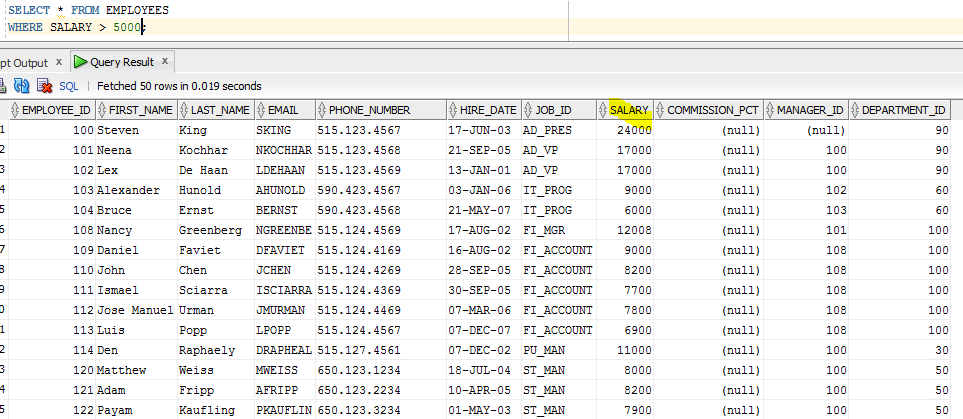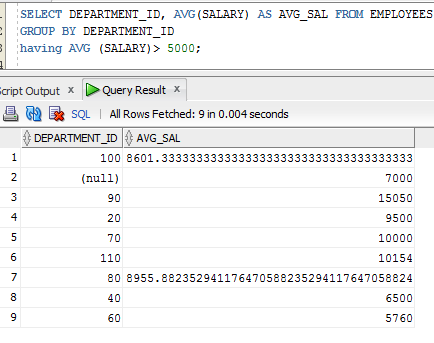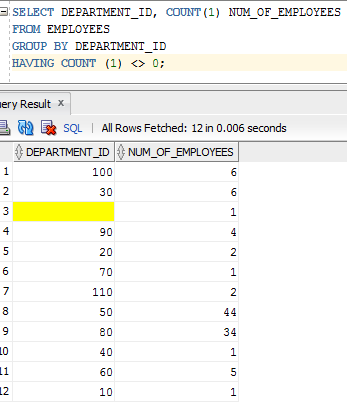# Aggregate Functions in SQL

### 1. What is the difference between WHERE clause and HAVING clause?

Both WHERE and HAVING clause are used to check the condition and have filters on the resultant data. But both are used in different contest. The WHERE clause is used with simple SELECT statement and to have subset of data from one or more tables. This clause will add the conditions and filter the resultant data set. A simple SELECT statement without WHERE clause will select all the data from the table/s. If we want to see only part or subset of data from the table, we will include WHERE clause.

The HAVING clause, though used in the SELECT statement, it is actually used to compare the aggregate values. i.e.; it can only compare the values of aggregate functions like SUM (), MAX (), MIN (), AVG (), and COUNT (). It cannot compare any simple conditions like WHERE clause does, while WHERE clause cannot compare aggregate values. Hence even though both of them are used for comparison, they are comparing different kinds of values.

Consider the example where we have to select the employees whose salary is more than 5000. Here we need to compare with the condition on SALARY column. Comparison will just check if the salary is more than 5000. Here no aggregate functionality is required. Hence we can use WHERE clause to compare if the salary of employee is more than 5000 in EMPLOYEES table.

```SELECT * FROM EMPLOYEES
WHERE SALARY > 5000;```Now consider the case where we have to find the departments whose employee’s average salary is more than 5000. Here we are not asked to compare the salary of employees in each department. Rather we have to find the average salary of employees in each department and then we have to compare if this average salary greater than 5000. That means we have to compare the result of GROUP BY function. Hence we have to use HAVING clause to compare the average salary of each department.

```SELECT DEPARTMENT_ID, AVG (SALARY) AS AVG_SAL
FROM EMPLOYEES
GROUP BY DEPARTMENT_ID
HAVING AVG (SALARY)> 5000;```We can note here in the query that GROUP BY is applied once all the data has been selected. That means, in order to apply group by on the departments here, we first have to select the data from the EMPLOYEES table. In our case above we first have to select all the departments and salary present in the EMPLOYEES table. If we have any WHERE clause in the query, then we have to apply that condition also to get the subset of data. Only then we can group the data to get the right result. If we apply GROUP BY clause before apply WHERE clause, then it will group the whole table’s data and we will not get correct result. Then we will be applying GROUP BY clause to get the result grouped into different departments and then we again apply condition on average salary. This is another reason why we have separate WHERE clause and HAVING clause. Comparing the aggregate values in the WHERE clause is same as comparing the values which are not fetched or calculated. These aggregate values are calculated only after WHERE clause is applied and GROUP BY function is applied.

### 2. Why do we need to use the same columns that are selected in the SELECT list in the GROUP BY Clause?

This is because, when we group the records based on some columns, and if we see the result without those columns, then we will not understand what is the result. If we see the result along with grouped columns, then we will get meaningful result and understand them too.

In below result, if we see AVG_SAL alone, then we will not understand what is it about. When we see DEPARTMENT_ID along with AVG_SAL, we will understand that it is average salary based on the departments. Therefore we need same columns in SELECT list as well as GROUP BY clause.### 3. Why do we need to have columns that are not part of GROUP BY Clause as part of aggregate function?

This is also same reason as above. If we have some columns in the SELECT list that are not part of GROUP BY clause, then it will change the requirement of query itself. Still if we need to include them in SELECT list but not in  GROUP BY clause, then we have use some aggregate function on them so that their value remains same but it will be displayed in the SELECT list.

### 4. Will fixing the error by adding the columns to Group By clause, result in correct output?

Not always. It always depends on the data and structure of the table.

### 5. In SQL, what’s the difference between the having clause and the group by statement?

Group by statement is used to group each value of particular columns and then calculate aggregated values. i.e.; when group by COLUMN_NAME is used, it first finds all the DISTINCT values from the column of the table. Then it finds the aggregate values based on this grouped column/s.  If we need to compare the value of this aggregated value, we cannot put it in WHERE clause. Instead we need to put HAVING clause to compare the aggregated values.

When we perform GROUP BY in a query, it first selects all the columns that are being selected from one or more tables by applying the conditions and filters in the WHERE clause. Once it gets this result, grouping of records are done using GROUP BY statement. Now this grouped record set maybe left without any aggregation or it may be aggregated to get sum, average, count etc. Now if we need to compare these aggregated values we cannot use WHERE clause. This is because WHERE clause has been already executed and after which we have got these aggregated values. Hence we need some other clause to compare these aggregated values. Hence we use HAVING clause to compare aggregated values like SUM, AVG, COUNT, MIN and MAX.

Consider the below query to find the number of employees in each department with at least 1 employee. That means we need to select all the employees from EMPLOYEES table first, then we need to group this result based on the DEPARTMENT_ID and find the count of employees in each department. Once this count is got, we need to find if the count is not equal to zero.

```SELECT DEPARTMENT_ID, COUNT (1) NUM_OF_EMPLOYEES
FROM EMPLOYEES
GROUP BY DEPARTMENT_ID
HAVING COUNT (1) <> 0;```Translate »